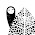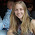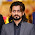## Saturday, 5 January 2013

### Filling an area under the graph

The following post is a portion of the D3 Tips and Tricks document which it free to download. To use this post in context, consider it with the others in the blog or just download the pdf  and / or the examples from the downloads page:-)
--------------------------------------------------------

Lines are all very well and good, but that's not the whole story for graphs. Some times you've just got to go with a fill.

Filling an area with a solid colour isn't too hard. I mean we did it by mistake back a few pages when we were trying to draw a line.

But to do it in a nice coherent way is fairly straight forward.

It takes three sections of code in much the same way that we drew our grid lines earlier it is done in three sections;
1. One in the CSS section to define what style the area will have.
2. One to define the functions that generate the area. And...
3. One to draw the area.
The end result will looks a bit like this;

### CSS for an area fill

This is pretty straight forward and only consists of two rules;
```.area {
fill: lightsteelblue;
stroke-width: 0;
}
```
Put them at the bottom of your <style> section.

The first one (fill: lightsteelblue;) sets the colour of our fill (and in this case we have chosen a lighter shade of the same colour as our line to match it) and the second one (stroke-width: 0;) sets the width of the line that surrounds the area to zero. This last rule is kind of important in making a filled area work well. The whole idea is that the graph is made up of separate elements that will compliment each other. There's the axes, the line and the fill. If we don't tell the code that there is no line surrounding the filled area, it will assume that there is one and add it in like this.
So what has happened here is that the area element has inherited the line property from the path element and surrounding the area is a 2px wide steelblue line. Not too pretty. Let's not go there.

### Define the area function

We need a function that will tell the area what space to fill. This is accessed from the d3.svg.area function (https://github.com/mbostock/d3/wiki/SVG-Shapes#wiki-area).

The code that we will use is as follows;
```var area = d3.svg.area()
.x(function(d) { return x(d.date); })
.y0(height)
.y1(function(d) { return y(d.close); });
```
I have placed it in between the axis variable definitions and the line definitions here;
```var yAxis = d3.svg.axis().scale(y)
.orient("left").ticks(5);
//  <==== Put the new code here!
var valueline = d3.svg.line()
.x(function(d) { return x(d.date); })
.y(function(d) { return y(d.close); });
```
You will notice it looks INCREDIBLY similar to the valueline function definition. That's because while the line definition describes drawing a line the connects a set of coordinates, I imagine the area definition describes drawing two lines that share the same x coordinates, but simultaneously draws two y coordinates, y0 and y1. Then when it's finished drawing the resultant shape, it fills it with the colour of your choosing.

So the only changes to the code are the addition of the 'y0' line and the renaming of the 'y' line 'y1'.

Here's a picture that might help explain;
As should be apparent, the top line (y1) follows the valueline line and the bottom line is at the constant 'height' value. Everything in between these lines is what gets filled. The function in this section describes the area.

### Draw the area

Now to the money maker.

The final section of code in the area filling odyssey is as follows;
```svg.append("path")
.datum(data)
.attr("class", "area")
.attr("d", area);
```
We should place this block directly after the domain functions but before the drawing of the valueline path;
```x.domain(d3.extent(data, function(d) { return d.date; }));
y.domain([0, d3.max(data, function(d) { return d.close; })]);
//  <== Area drawing code here!
svg.append("path")
.attr("class", "line")
.attr("d", valueline(data));
```
This is actually a pretty good idea to put it there since the various bits and pieces that are drawn in the graph are done so one after the other. This means that the filled area comes first, then the valueline is layered on top and then the axes come last. This is a pretty good sequence since if there are areas where two or more elements overlap, it might cause the graph to look 'wrong'.

For instance, here is the graph drawn with the area added last.
You should be able to notice that part of the valueline line has been obscured and the line for the y axis where it coincides with the area is obscured also.

Looking at the code we are adding here, the first line appends a path element (svg.append("path")) much like the script that draws the line.

The second line (.datum(data)) declares the data we will be utilising for describing the area and the third line (.attr("class", "area")) makes sure that the style we apply to it is as defined in the CSS section (under 'area').
The final line (.attr("d", area);) declares “d” as the attributer for path data and calls the 'area' function to do the drawing.

And that's it!

### Filling an area above the line

Pop Quiz:
How would you go about filling the area ABOVE the graph?

Now it might sound a little trite, but believe it or not, this could come in handy. For instance, what if you want to highlight an area that was too high and an area that was too low for a line of data on a graph with an area in the centre where a projected 'normal' set of values should be present?

In this instance, you could fill the lower area as has been demonstrated here, and with a small change you can fill another area with a solid colour above another line.

How is this incredible feat achieved?

Well, remember the code that defined the area?
```var area = d3.svg.area()
.x(function(d) { return x(d.date); })
.y0(height)
.y1(function(d) { return y(d.close); });
```
All we have to do is tell it that instead of setting the y0 constant value to the height of the graph (remember, this is the bottom of the graph) we will set it to the constant value that is at the top of the graph. In other words zero (0).
```.y0(0)
```

That's it.
Now, I'm not going to go over the process of drawing two lines and filling each in different directions to demonstrate the example I described, but this provides a germ of an idea that you might be able to flesh out :-).

The above description (and heaps of other stuff) is in the D3 Tips and Tricks document that can be accessed from the downloads page of d3noob.org.

1.Thank you for explanation!

2.Can't find a link to .tsv data file, could you please provide some sample data set?

1.Hi, it should be in the zip file of examples (https://dl.dropbox.com/u/101577503/d3noob.zip) (in the data directory) which is on the the downloads page (http://www.d3noob.org/p/d3noob-downloads.html). Sorry the examples are a bit of a legacy of a few different publishing methods. Hopefully I'll have an opportunity to incorporate them all into the book download (a few are there now) and into the examples on bl.ocks.org (http://bl.ocks.org/d3noob). Cheers.

3.This is great! It is really hard for me to find well explained d3 tutorials. Thanks a lot!

4.Thanks for the nice article. I ran across your post because I need a special fill. Suppose on your area graph, we have a line connecting top left corner with bottom right corner and we want to fill the area above the line and below the curve, but not above the curve and below the line. Is it possible? How?

5.Hi. This article helped me greatly. I am new to d3 and such examples help me a lot. I don't know if this would be the right place to ask the question but is it possible to shade the area with different colours ( for example > 10, should be shaded green, 10 to 5 should be shaded yellow and <5 should be shaded red ?

Thanks for your time and reply !

1.Good question (and sorry for the delay in replying) Yes, absolutely. In fact if you go to this page (http://www.d3noob.org/2013/01/applying-colour-gradient-to-area-fill.html) there is an example of exactly what you're looking for. Probably best to download a copy of thee entire book (It's free) from Leanpub (https://leanpub.com/D3-Tips-and-Tricks) and then you can download the code really simply as well.

6.Hi! I tried copying the code from your book for this but all I get is a blank page (in Chrome and Internet Explorer).

1.Hmm... I'm not sure what the problem might be, but there is always a possibility that there are some character encoding issues. I would recommend downloading the code examples that come with the book (from Leanpub) and using the appropriate file from there. That is tested and works :-).

7.Thanks for this nice explaination.

8.Thanks for full explanation